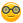# Does anyone know why this code is not printing at the end?

selected = 6
num1 = input("First number:")
if num1 == selected:
print("Hi")

I entered 6 in the input

Python 3 stores the input as 'string' type, So when you type 6, it is actually being stored as '6' of type string. And therefore 6 is not equal to '6'.

num1 = int(input("First number:"))

answered Mar 12 by (170 points)

you write     print("Hi")    rong

write             printf("Hi");     right

commented Mar 12 by (240 points)
This Isn't C++, Idiot.
+1 vote
string "6" !=6

so u have to use int(input())
+1 vote
answered Mar 12 by (200 points)

input we give while run programm is string not intselected = 6
num1 =int( input("First number:"))
if num1 == selected:
print("Hi")